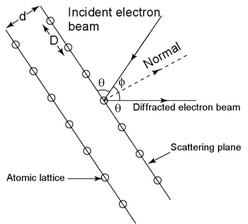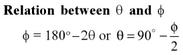# Origin Of Quantum Mechanics MCQ Level – 1

## 10 Questions MCQ Test Topic wise Tests for IIT JAM Physics | Origin Of Quantum Mechanics MCQ Level – 1

Description
This mock test of Origin Of Quantum Mechanics MCQ Level – 1 for Physics helps you for every Physics entrance exam. This contains 10 Multiple Choice Questions for Physics Origin Of Quantum Mechanics MCQ Level – 1 (mcq) to study with solutions a complete question bank. The solved questions answers in this Origin Of Quantum Mechanics MCQ Level – 1 quiz give you a good mix of easy questions and tough questions. Physics students definitely take this Origin Of Quantum Mechanics MCQ Level – 1 exercise for a better result in the exam. You can find other Origin Of Quantum Mechanics MCQ Level – 1 extra questions, long questions & short questions for Physics on EduRev as well by searching above.
QUESTION: 1

### If first excitation potential of a hydrogen-like atom is V eV, then the ionization energy of this atom will be :

Solution:

First excitation energy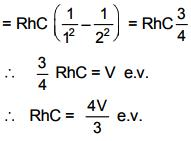QUESTION: 2

### Linear momenta of a proton and an electron are equal. Relative to an electron

Solution:

If pe = pp,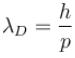therefore, de-Broglie wavelength of proton and electron are equal.
The correct answer is: de-Broglie wavelength of proton and electron are equal

QUESTION: 3

### The wavelength of a photon and the de-Broglie wavelength of an electron and uranium atom are identical. Which one of them will have highest kinetic energy

Solution: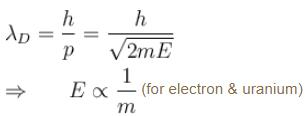for photon & electron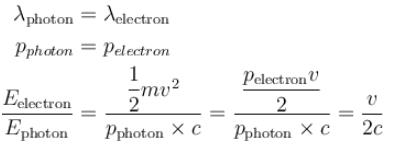QUESTION: 4

The energy E and momentum p of photon is given by E = hv and  p = h/λ, the velocity of photon will be :

Solution:

E = hc/λ     put c = v
and  p = h/λ
E = pv
⇒ v = E/p

QUESTION: 5

In the figure six lines of emission spectrum are shown. Which of them will be absent in the absorption spectrum.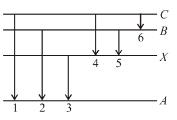Solution:

The photon’s with energies equal to that required for upward transition A → X, A → B and A → C would be absorbed, these photons shall be absent from absorption spectrum.

The correct answer is: 1, 2, 3

QUESTION: 6

A charge particle q0 of mass m0 is projected along the y-axis at t = 0 from origin with a velocity V0. If uniform electric field E0 also exists along the x-axis, then the time at which de-Broglie wavelength of the particle becomes half of the initial value is :

Solution:

Initial debrogle wavelength of the charge particle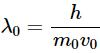After any time t,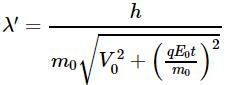Suppose after time t,  λ becomes half of the initial value i.e.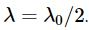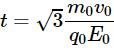QUESTION: 7

What voltage must be applied to an electron microscope to produce electrons of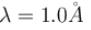Solution: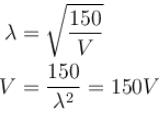The correct answer is: 150 Volt

QUESTION: 8

In X-rays production an electron accelerated with voltage V strikes a metal target. For which of the following voltages X-rays of minimum wavelength will be produced :

Solution:

λ will be minimum for maximum accelerated voltage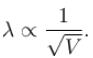The correct answer is: 40 kV

QUESTION: 9

In the photoelectric, if we use a monochromatic light, the I-V curve is as shown. If work function of the metal is 2eV, estimate the power of light used. (Assume efficiency of photo emission = 10–3 % i.e. number of photoelectrons emitted are 10–3 % of number of photons incident on metal.)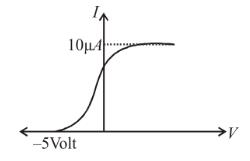Solution:

The energy of incident photons is given by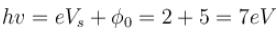(Vs is stopping potential and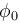is work function)
Saturation current =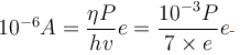(η is photo emission efficiency)
∴ P = 7mW